# Hex, Bugs and More Physics | Emre S. Tasci

a blog about physics, computation, computational physics and materials…

## A (Lame) Proof of the Probability Sum Rule

### December 21, 2007 Posted by Emre S. Tasci

Q: Prove the Probability Sum Rule, that is: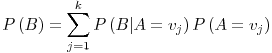(where A is a random variable with arity (~dimension) k) using Axioms:and assuming: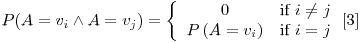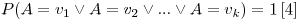We will first prove the following equalities to make use of them later in the actual proof: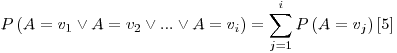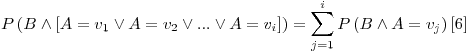Proof of :

Using , we can write: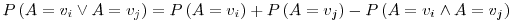where, from the assumption , we have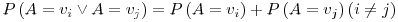Using this fact, we can now expand the LHS of  as: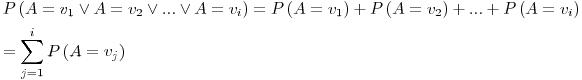Proof of :

Using the distribution property, we can write the LHS of  as: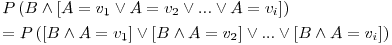from , it is obvious that:then using , we can rearrange and rewrite the LHS of  as: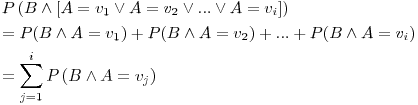Final Proof :

Before proceeding, I will include two assumptions about the Universal Set (this part is the main reason for the proof to be lame by the way).

Define the universal set U as the set that includes all possible values of some random variable X. All the other sets are subsets of U and for an arbitrary set A, we assume that:Furthermore, we will be assuming that, a condition that includes all the possible outcomes(/values) for a variable A is equivalent to the universal set. Let A have arity k, then:You can think of the condition (U) as the definite TRUE 1.

Now we can begin the proof of the equalityusing the Bayes Rule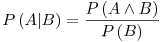we can convert the inference relation into intersection relation and rewrite RHS as: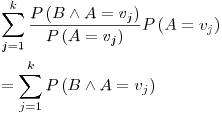using , this is nothing but: